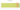# د.أ.‏

## Jordanian Dinar

Since 1950, Jordan has used the Jordanian dinar as its official currency. One dinar is equal to ten dirhams, one hundred qirsh, or one thousand fulus. The US dollar is the reference point. In place of the Jordan Currency Board, the Central Bank of Jordan started operations in 1964 and took over as the country's sole issuer of currency. Along with the Israeli shekel, the Jordanian dinar is a commonly accepted currency in the West Bank.

#### how has the value of the currency changed in the last year?## USD 1 = JOD 0.7096

How much is10 US dollars worth inJordanian dinars?
At the current exchange rate, 10 US dollars is worth 7.1 Jordanian dinars
How much is50 US dollars worth inJordanian dinars?
At the current exchange rate, 50 US dollars is worth 35.48 Jordanian dinars
How much is100 US dollars worth inJordanian dinars?
At the current exchange rate, 100 US dollars is worth 70.96 Jordanian dinars
How much is500 US dollars worth inJordanian dinars?
At the current exchange rate, 500 US dollars is worth 354.8 Jordanian dinars
How much is2,000 US dollars worth inJordanian dinars?
At the current exchange rate, 2,000 US dollars is worth 1,419.2 Jordanian dinars
How much is10 US dollars worth inJordanian dinars?
At the current exchange rate, 10 US dollars is worth 7.1 Jordanian dinars
How much is50 US dollars worth inJordanian dinars?
At the current exchange rate, 50 US dollars is worth 35.48 Jordanian dinars
How much is100 US dollars worth inJordanian dinars?
At the current exchange rate, 100 US dollars is worth 70.96 Jordanian dinars
How much is500 US dollars worth inJordanian dinars?
At the current exchange rate, 500 US dollars is worth 354.8 Jordanian dinars
How much is2,000 US dollars worth inJordanian dinars?
At the current exchange rate, 2,000 US dollars is worth 1,419.2 Jordanian dinars